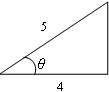# Cosine function

To use this function, choose Calc > Calculator.

The cosine of an acute angle of a right triangle is the ratio of the adjacent leg to the hypotenuse (the longest side, opposite the right angle), measured in radians.The cosine of angle θ is the ratio 4/5, or 0.8.

The cosine of θ can also be defined more generally as the x-coordinate of the point on the unit circle that is located at angle θ from the origin (measured counterclockwise from the positive x-axis).